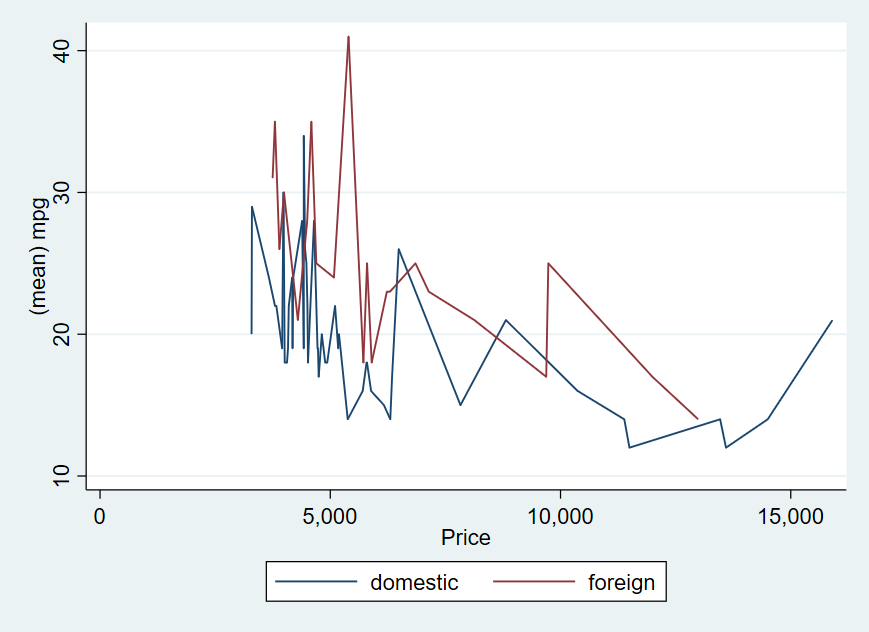Search

# Graphing the mean of a variable by other variables

In this post, I will show you how to graph the mean of a variable by other variables. The dataset I used is automobile dataset. An worked example is shown below:It shows the mean of variable mpg by different prices. The commands I used in a do-file are as below:

```sysuse auto, clear
collapse (mean) mpg , by(for price)
twoway                      ///
(line  mpg price if for==0) ///
(line  mpg price if for==1), legend( label(2 "foreign" ) label(  1 "domestic" ))```

You may find more useful information in the book A Visual Guide to Stata Graphics